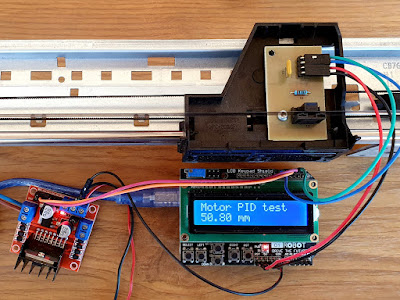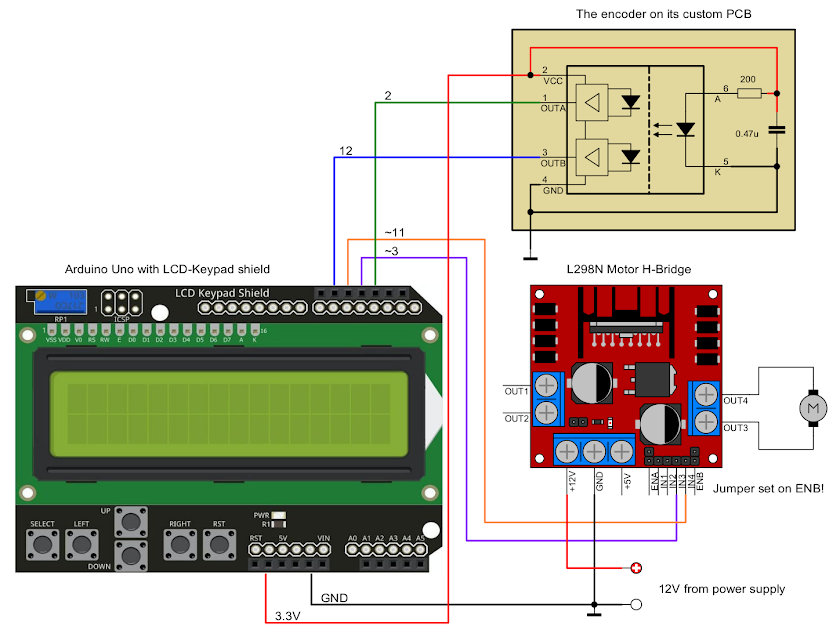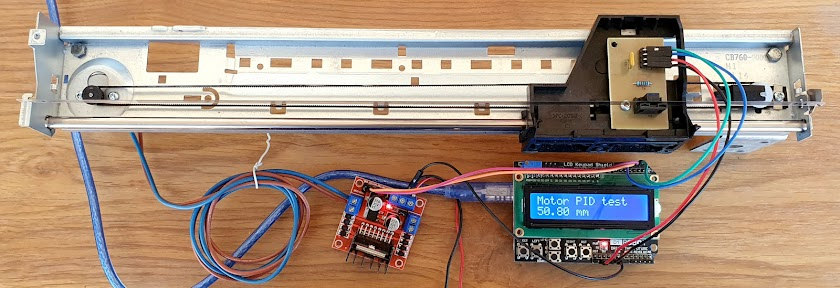### PID control for DC motor with optical encoder

How to move a sliding cartridge unit from an inkjet printer using a PID controlled DC motor with optical encoder feedback

In the previous post I gathered information about a cartridge slide unit taken from an old inkjet printer. As I found out, the printer used a common DC motor to move the cartridges on X axis, however with the addition of an optical encoder with strip, the cartridges could perform precise movements. So, I want to interface this to Arduino. Original printer electronics such as motor drivers were of no use (proprietary ICs without public datasheets).

I had to drive the motor with a L298N H-bridge module and for the encoder I built a PCB which replaced the original one used in printer. The software is not as simple as you may think. I just can't turn on motor until the reading of encoder equals the desired position. Suddenly stopping the motor will not result in a sudden stop of the sliding block. Due to inertia, it continues to move a bit, even with the motor electrically shorted. The proper approach requires a PID (proportional-integral-derivative) control algorithm which adjusts motor speed using PWM.The motor with optical encoder wired to Arduino

## Wiring

Let's start with the easy stuff: wiring and encoder testing. I'm not saying PID is difficult when you have Arduino libraries. But tweaking the PID parameters for the best results took me a while. I used an Arduino Uno board fitted with the LCD-Keypad shield. That's optional, you may use any Arduino board which has 2 PWM outputs and one interrupt pin available.Arduino motor PID controller schematic

I've seen people using L298N with PWM in two ways. Using regular digital pins for H-bridge and a single PWM pin wired to enable input is wrong. When PWM output is low, the H-bridge is disabled and the motor axis can be easily rotated by external forces. The right way is to apply PWM at the H-bridge input. Two PWM outputs are required because the motor must be able to rotate in either direction.

L298N is powered from 12 V and so is the motor. Without available datasheet, I powered the encoder with 3.3 V and it seems to behave properly at this voltage. Arduino gets is power from USB port. One encoder output must be wired to an interrupt capable pin because this is the way used to count strip lines. The H-bridge inputs must be wired to PWM capable pins on Arduino. Since I used that shield, I had available 3 and 11 for PWM. Pin 12 is used for the other output of the encoder.The full sliding unit interfaced to Arduino

## Testing

Let's write the code for the encoder. I've wired one of its outputs to pin D2 to use the interrupt on this pin. Reading the position becomes very simple. First, I set up the interrupt:

attachInterrupt(0, processEncoder, FALLING);

Then I write the function processEncoder() like this:

void processEncoder() {
if (PINB & 0x10) encoderPos++;
else encoderPos--;
}

Bit 4 (0x10) of PORTB is actually pin D12 of Arduino Uno and other ATmega328 Arduino boards. I used direct port reading to spare some CPU cycles because interrupt functions should return as fast as possible. In the case of my sliding unit, the encoder block can't physically reach any of the extremities of the strip. So, there's no way of performing some sort of homing without an additional endstop switch. But, if the encoder could reach an area of the strip without lines, additional code would be required in the interrupt routine to detect this and use it as a "landmark" for finding the absolute position.

Anyway, by now I can display the block relative position using this code:

void loop() {
// check if movement occurred and display distance
float currentPos = encoderPos / stripLPI * 25.4;
if (currentPos != lastKnownPos) {
lcd.setCursor(0, 1);
lcd.print(currentPos);
lcd.print(" mm        ");
lastKnownPos = currentPos;
}
}

Besides this, let's add a function to move the block (turn the motor on):

void moveMotor(int pwm) {
if (pwm > 0) {
analogWrite(motorDirR, pwm);
digitalWrite(motorDirL, LOW);
}
else {
pwm = abs(pwm);
analogWrite(motorDirL, pwm);
digitalWrite(motorDirR, LOW);
}
}

Use a positive argument (between 1 and 255) and the motor will move to the right. Use a negative argument (between -255 and -1) and the motor will move to the left. This code is written for compatibility with the PID output.

It seems we got everything ready for the implementation of the PID controller. Not quite. At low PWM factors the motor will not move at all. At high PWM factors the motor will move with nearly identical and too much speed. Tuning the PID becomes difficult in these situations. So, I wrote a function that tests the motor response to PWM. Basically, this function attempts to move the motor while increasing PWM. It displays the PWM at which the motor starts rotating. I chose to display the PWM at which the sliding block moved 1/30" in 0.5 seconds (that's nearly 1.7 mm/s). It continues to increase the PWM factor until the block performs a 3" movement in 0.5 seconds (152 mm/s).

At high speeds, you will see the block moving more than 3 inches because of inertia. That's why the function waits for another 0.5 seconds before trying the next PWM factor. I wrote a test sketch that will move the motor back and forth and calculate movement speed. The speed is plotted over serial port, while the PWM at which the motor starts moving and the PWM at which the motor moves fast enough are displayed on 16x2 LCD. The sketch is available at the end of this post. Move the sliding block with your hand at the approximate center of the unit, then upload this sketch. The sliding block will start moving with increasing speed and distance. When the test ends, this is displayed on LCD (actual PWM factors may be different with other motors):

Move start: 58
Test stop:  84

These are my output values and they are slightly different at each test repetition. Do not touch or block the movement while the test is running. Also note that these values change depending on load and friction. When the test is done, you are free to move the block by hand. Current relative position in mm will be displayed.

## PID Controller

It's fairly easy to implement the algorithm using existing libraries. The PID controller takes as arguments the current position (input) and the position we want to go (setpoint). Based on difference between these (the "error") it computes a PWM factor required to move the motor to the desired position (output). As the motor moves the block, the new position (input) is passed to PID controller and when it gets closer to the desired position (setpoint), the PWM factor (output) decreases to allow precise stopping. The algorithm response to the difference between setpoint and input and to the variation of this difference over time is controlled by three parameters: Kp (sets the proportionality with the error), Ki (tends to compensate the error by integrating past values over time) and Kd (seeks to reduce the error on future outputs).

Let's get to the code. This is how I declared the PID controller:

double kp = 5, ki = 0.1, kd = 0.05;
double initialPos = 0, /* where we are */
pwmFactor = 0, /* the "amount" of action we need to take (pulse width here) */
desiredPos = 0; /* where we want to be */

PID motorPID(&initialPos, &pwmFactor, &desiredPos, kp, ki, kd, DIRECT);

PID produces an output that can be bound between any limits we choose. Remember the PWM test? The effective PWM was in my case between 58 and 84. I'll extend the range a bit to 55-85. We must configure PID to output these values. In setup():

  /* Configure PID controller */
motorPID.SetMode(AUTOMATIC);
motorPID.SetSampleTime(10);
motorPID.SetOutputLimits(-30, 30);

Negative output is required to know when to move backwards. I set it to output values up to 30 = 85-55. Next, the moveMotor(int pwm) must be modified to pass only 55..85 PWM to the motor.

void moveMotor(int pwm) {
if (pwm > 0) {
pwm = pwm + 55;
analogWrite(motorDirR, pwm);
digitalWrite(motorDirL, LOW);
}
else {
pwm = abs(pwm) + 55;
analogWrite(motorDirL, pwm);
digitalWrite(motorDirR, LOW);
}
}

The PID output (-30..30) can now be used as argument for this function. PID controllers process input data repeatedly (note the SetSampleTime(10) call which sets PID sampling time to 10 milliseconds). That's why we must re-compute the output periodically. I wrote the next function which gets called in loop():

void motorAction() {
initialPos = encoderPos;
if (desiredPos == initialPos) {
// do nothing
digitalWrite(motorDirL, LOW);
digitalWrite(motorDirR, LOW);
return;
}

motorPID.Compute();
moveMotor(pwmFactor);
}

It does nothing if position is correct. Otherwise it computes the PWM using PID and moves the sliding block. Note that I could have skipped that initial position check. If desired position and actual position match, PID will set PWM to 0.

## Final considerations

• Adjust PID constants for best results. Kp will proportionally increase moving speed.
• Check your wiring if the motor goes to one end and tends to go even further. Switch either encoder pins either H-bridge inputs or output.
• If the motor oscillates back and forth it means that it moves too fast and each new PID computing finds it past the setpoint. The meaning of "moves too fast" can be either too much speed (see Kp and maximum PWM factor) either slow PID (see sampling time).
• If you get oscillations, avoid reducing sampling time. It's already very low.
• PID algorithm in this library works with double type variables. We don't usually need so much precision, yet internal library computing over time is most accurate with such variables.
• Especially for this application, the real position must be able to equal desired position to get 0 output and stop the motor. Otherwise it will just vibrate near the set position, back and forth one strip line. Do not pass impossible positions which cannot be achieved with current strip LPI.
• The sketch below is for Arduino Uno with LCD-Keypad shield. Use up/down buttons to set step size and left/right to move sliding bock one step.
• Try to move the motor axis (sliding block) by hand. It will always (or it should) go back to its set position.